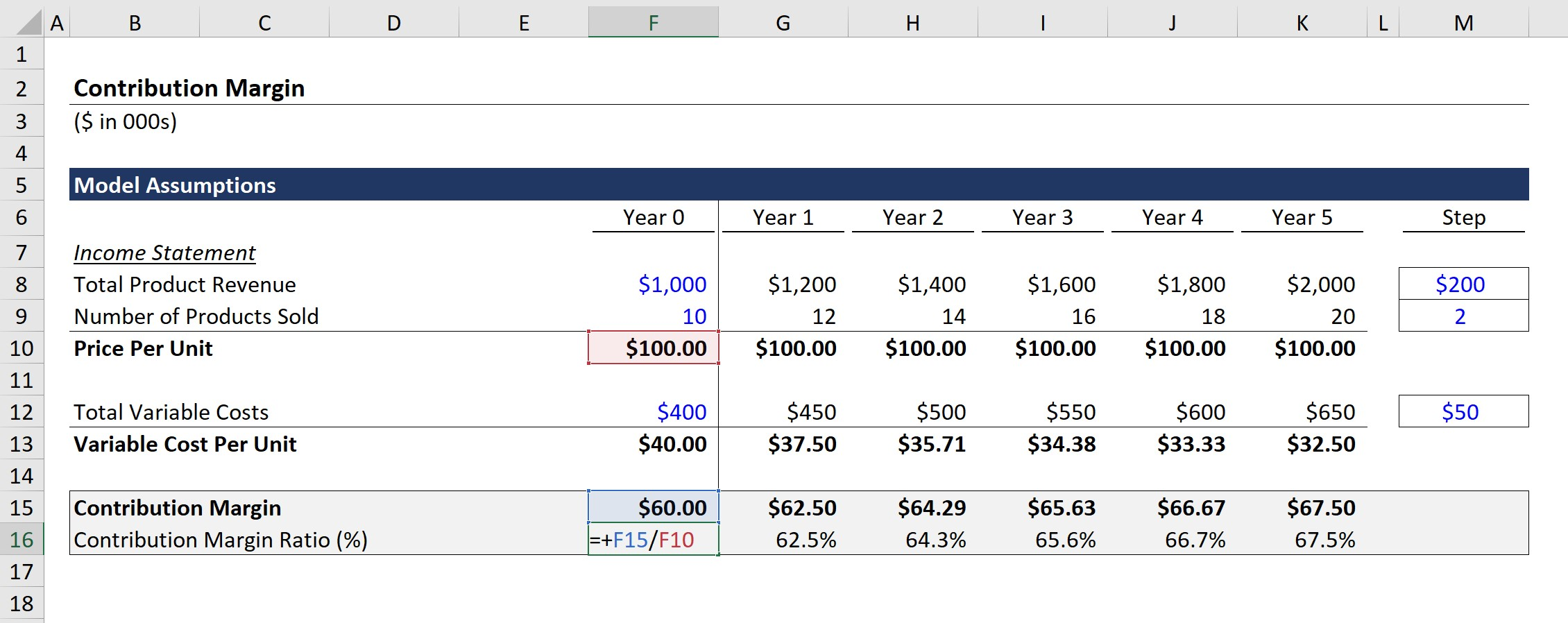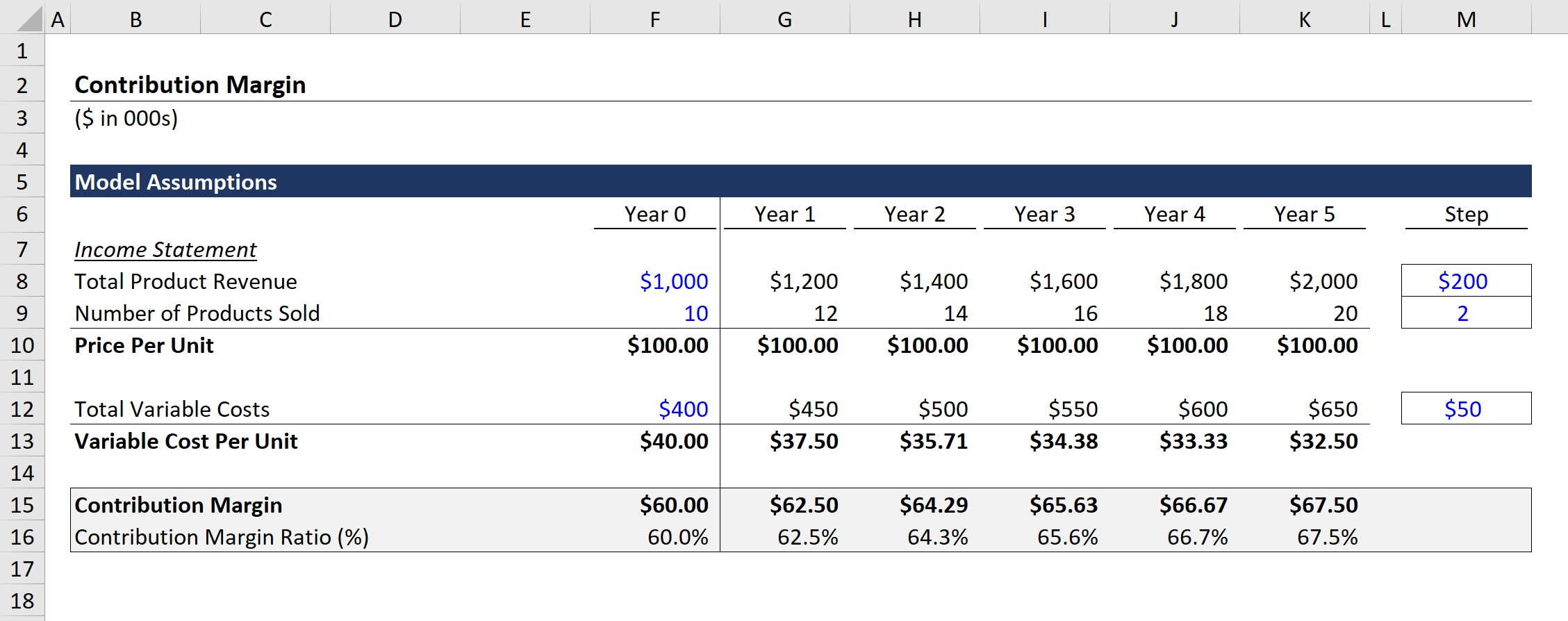# Contribution Margin

Guide to Understanding the Contribution Margin• What does the contribution margin measure?
• What is the formula used to calculate the contribution margin?
• How is the contribution margin related to the break-even concept?
• Why might the contribution margin be preferred over the gross margin metric?

## Contribution Margin Definition

While there are various profitability metrics – ranging from the gross margin down to the net profit margin – the contribution margin (CM) metric stands out for the analysis of a specific product/service.

The contribution margin is the profits generated after variable costs have been deducted – i.e. how much revenue exceeds the associated variable costs.

Instead of looking at the profitability of a company on an overall basis with all products grouped together, the CM enables margin analysis on an individual product line basis.

In particular, the use-case of the metric tends to be most applicable for setting prices appropriately.

If the CM is too low, the current price point may need to be reconsidered.

In such cases, the price of the product would either be:

• Increased: The pricing of the product line could be raised to contribute towards more profitability, as the current profit margins are unsustainable.
• (or) Discontinued: The product offering could be discontinued if there is insufficient customer demand after a potential price increase.

Additionally, the assessment of the CM can help determine the optimal pricing by assessing the impact each change would have on revenue and profitability levels.

Given how the CM examines the product-level breakdown of each dollar that comes in and how it contributes to generating profit, the break-even point cannot be calculated without determining the CM.

For companies seeking to obtain a sustainable long-term competitive advantage, it’s important to focus on identifying the products with the highest CMs in order to maximize potential profits.

## Contribution Margin Formula

The calculation of the metric is relatively straightforward, as the formula consists of revenue minus variable costs.

###### Formula
• Contribution Margin = Revenue – Variable Costs

Just as a quick review, a company’s variable costs are directly associated with revenue and fluctuate based on production volume, whereas fixed costs remain unchanged regardless of production volume.

• Variable Costs: e.g. Purchase of Inventory/Materials, Direct Labor, Shipping Costs
• Fixed Costs: e.g. Utilities, Rent, Insurance, Equipment

All else being equal, the greater the CM of each product, the more profitable the company is going to be, with more cash available to meet other expenses.

The distinction pertains to the concept of scalability, as companies with higher variable costs tend to bring in fewer profits since the direct costs increase (and can offset) the growth in revenue.

## Contribution Margin Ratio Formula

Furthermore, to perform a more detailed analysis on either a quarterly or year-over-year (YoY) basis – or comparisons to comparable companies in the same industry – the CM can be divided by revenue to get to the CM ratio.

###### Formula
• CM Ratio = (Revenue – Variable Costs) ÷ Revenue

To go through a simple example, let’s say there’s an e-commerce company selling t-shirts for \$25.00 with variable costs of \$10.00 per unit.

The formula used to calculate the CM would be as follows:

• \$25.00 – \$10.00 = \$15.00

Next, the CM ratio can be calculated using the following formula:

• \$15.00 ÷ \$25.00 = 60%

The 60% CM ratio means that the contribution margin for each dollar of revenue generated is \$0.60.

###### Contribution Margin vs Gross Margin

One common area of misunderstanding is related to the difference between the CM and the gross margin (GM).

The gross margin metric – which is calculated as gross profit divided by revenue – measures the amount of revenue remaining once all direct costs (i.e. cost of goods sold, or COGS) have been subtracted.

When it comes to the CM, the intent behind the calculation is similar but can be analyzed more in-depth on a per-item/per-product profitability level.

On the other hand, the gross margin represents the total profit metric inclusive of all products/services offered by the company.

## Contribution Margin Calculator – Excel Template

We’ll now move to a modeling exercise, which you can access by filling out the form below.Submitting ...

## Contribution Margin Model Assumptions

As the first step, we’ll begin by listing out the model assumptions for our simple exercise.

As of Year 0, the first year of our projections, our hypothetical company has the following financials.

###### Model Assumptions

Income Statement Data (Year 0)

• Total Product Revenue: \$1m
• Number of Products Sold: 10k
• Total Variable Costs: \$400k

Using the provided data above, we can calculate the price per unit by dividing the total product revenue by the number of products sold.

• Price Per Unit = \$1m ÷ 10k = \$100.00

Similarly, we can then calculate the variable cost per unit by dividing the total variable costs by the number of products sold.

• Variable Cost Per Unit = \$400k ÷ 10k = \$40.00

For the rest of the forecast period – i.e. Year 1 and onward – we’ll just assume that each line item will grow by the following:

• Total Product Revenue: Increase by +\$200k / Year
• Number of Products Sold: Increase by +2k / Year
• Total Variable Costs: Increase by +\$50k / Year

If we fill out the “Price Per Unit” and “Variable Cost Per Unit” for each projected year, from Year 0 to Year 5, the figures change from:

• Price Per Unit: No Change (\$100.00)
• Variable Cost Per Unit: \$40.00 → \$32.50

For this section of the exercise, the key takeaway is that the CM requires finding the revenue from the sale of a specific product line, along with the specific variable costs.

In effect, the process can be more difficult in comparison to a quick calculation of gross profit and the gross margin using the income statement, yet is worthwhile in terms of deriving product-level insights.

## Contribution Margin Example Calculation

We’ll next calculate the contribution margin and CM ratio in each of the projected periods in the final step.

To calculate the CM, we simply deduct the variable cost per unit from the price per unit. For instance, in Year 0, we use the following formula.

• \$100.00 – \$40.00 = \$60.00

Next, the CM ratio can be calculated by dividing the amount from the prior step by the price per unit, as shown in the screenshot below.In Year 0, if we divide the CM by the price per unit, we get the following CM margin %:

• \$60.00 ÷ \$100.00 = 60.0%

Here, we are calculating the CM on a per-unit basis, but the same values would be obtained if we had used the total figures instead.

From Year 0 to Year 5, the following changes occur:

• CM: \$60.00 → \$67.50 CM
• CM Ratio: 60.0% → 67.5%

Note that the overarching objective of calculating the contribution margin is to figure out how to improve operating efficiency by lowering each product’s variable costs, which collectively contributes to higher profitability.

The profitability of our company likely benefited from the increased contribution margin per product, as the contribution per dollar increased from \$0.60 to \$0.68.Step-by-Step Online Course

#### Everything You Need To Master Financial Modeling

Enroll in The Premium Package: Learn Financial Statement Modeling, DCF, M&A, LBO and Comps. The same training program used at top investment banks.Inline FeedbacksLearn Financial Modeling Online

Everything you need to master financial and valuation modeling: 3-Statement Modeling, DCF, Comps, M&A and LBO.

X

The Wall Street Prep Quicklesson Series

7 Free Financial Modeling Lessons

Get instant access to video lessons taught by experienced investment bankers. Learn financial statement modeling, DCF, M&A, LBO, Comps and Excel shortcuts.